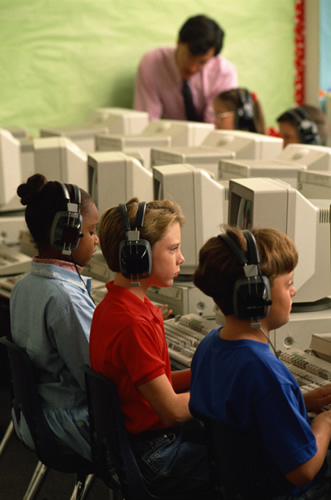•LEARNING RESOURCES

AAA MATH : Has numerous interactive Math lessons and practice problems.

MATH IS FUN : Makes math enjoyable with lesson, quiz, practice problems and more for all grade levels.

MULTIPLICATION PRACTICE : Fun way to learn addition, subtraction, multiplication and division

STARFALL FOR READING AND MATH :  Great websites for phonics, reading, math, rhymes, games etc.,

BRAINPOP : For videos on all subjects, social emotional learning and fun games

READ THEORY : For leveled texts, helps with reading and reading comprehension

MATH PRACTICE : Fun way to learn Math